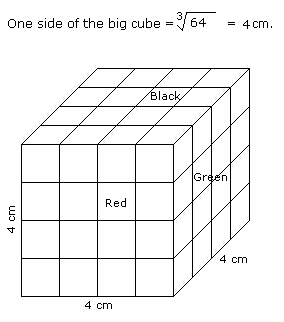# Verbal Reasoning - Cube and Cuboid - Discussion

Discussion Forum : Cube and Cuboid - Cube and Cuboid 5 (Q.No. 5)
Directions to Solve

The following questions are based on the information given below:

All the opposite faces of a big cube are coloured with red, black and green colours. After that is cut into 64 small equal cubes.

5.
How many small cubes are there whose at the most two faces are coloured ?
48
56
28
24
Explanation:Number of small cubes having two faces coloured = 8 + 8 + 4 + 4 = 24

and Number of small cubes having only one face coloured = 4 x 6 = 24

and Number of small cubes having no face coloured = 4 + 4 = 8

Therefore, total number of small cubes whose at the most two faces are coloured = 24 + 24 + 8 = 56.

Discussion:
3 comments Page 1 of 1.

Nitin said:   5 years ago
Why we need to count the cubes whose faces have no colour? When it's mentioned that at the most two sides "coloured".

Maximum faces colored for any cube is '3' in the given scenario.

It's better to subtract '3' faces colored cubes from total cubes then we will get the number of cubes that are at most two faces colored.

i.e. (4^3)-8 = 64-8= 56.
(1)